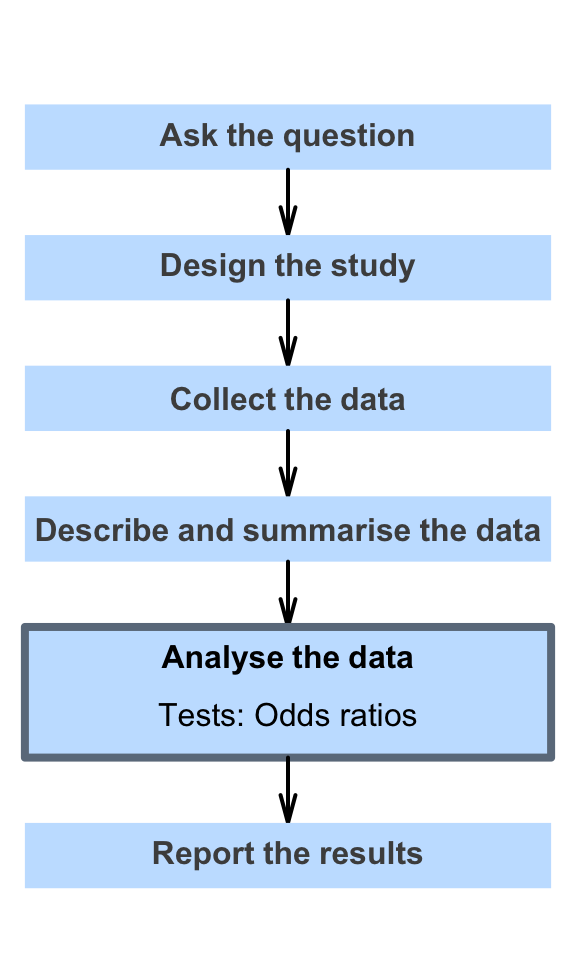# 31 Hypothesis tests for comparing odds

So far, you have learnt to ask a RQ, identify different ways of obtaining data, design the study, collect the data describe the data, summarise data graphically and numerically, understand the tools of inference, and to form confidence intervals.

In this chapter, you will learn about hypothesis tests for odds ratios. You will learn to:

• conduct hypothesis tests for an OR (i.e., comparing two proportions, or comparing two odds), using chi-square tests using jamovi and SPSS output.
• determine whether the conditions for using these methods apply in a given situation.